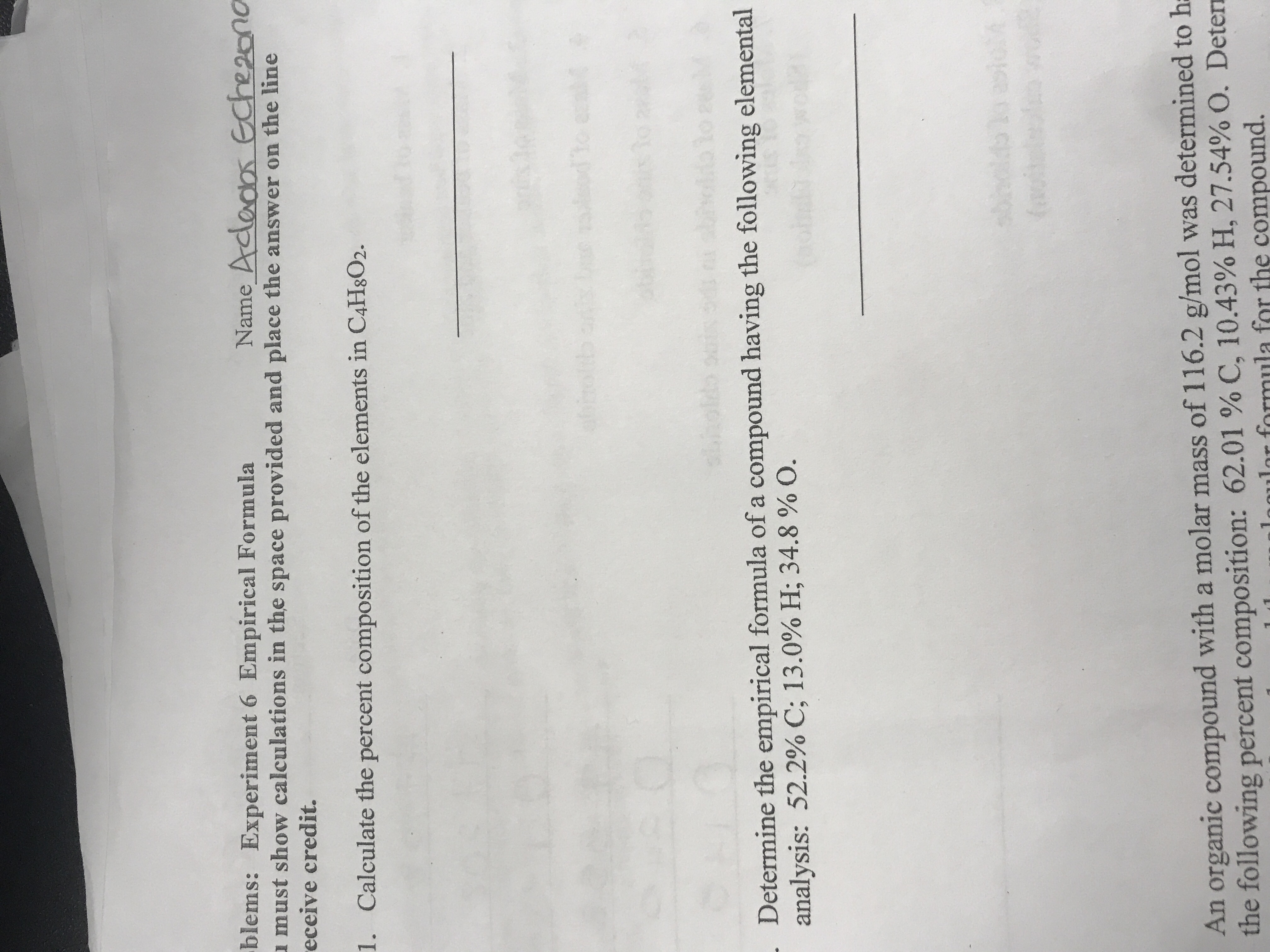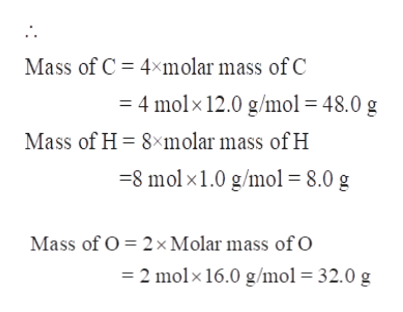# blems: Experiment 6 Empirical Formulamust show calculations in the space provided and place the answer on the lineeceive credit.Name Adados Eche2onoCalculate the percent composition of the elements in C4H8O2.1.to erni tebhod*fos11491Determine the empirical formula of a compound having the following elementalanalysis: 52.2% C; 13.0 % H; 34.8 % O.MM51An organic compound with a molar mass of 116.2 g/mol was determined to hthe following percent composition: 62.01 % C, 10.43 % H, 27.54% O. Deter1nmulor formula for the compound.

Question
16 viewshelp_outlineImage Transcriptioncloseblems: Experiment 6 Empirical Formula must show calculations in the space provided and place the answer on the line eceive credit. Name Adados Eche2ono Calculate the percent composition of the elements in C4H8O2. 1. to erni t ebho d *fo s1 14 91 Determine the empirical formula of a compound having the following elemental analysis: 52.2% C; 13.0 % H; 34.8 % O. MM 51 An organic compound with a molar mass of 116.2 g/mol was determined to h the following percent composition: 62.01 % C, 10.43 % H, 27.54% O. Deter 1nmulor formula for the compound. fullscreen
check_circle

Step 1

The percent composition of elements in C4H8O2 is to be calculated.

Step 2

The percent composition of the elements can be determined from the formula of the compound.

1. First, calculate the mass of each element in 1mole of compound.
2. Calculate the total molar mass of the compound.
3. Then percent composition of each element can be calculated by dividing the mass of each element by the molar mass of the compound and then multiplied by 100.
Step 3

Now calculate the mass of C, H and O in 1 mole of C4H8O2-

1 mole of C4H8O2 consists of 4 moles of carbon, 8 moles of Hydrogen and 2 moles of oxygen.

Thus, in C4H8O2

Mass of C = 4×molar mass of C

Mass of H = 8×molar mass of H

Mass of O = 2&...help_outlineImage TranscriptioncloseMass of C 4 molar mass of C =4 molx 12.0 g/mol = 48.0 g Mass of H 8xmolar mass of H -8 mol x1.0 g/mol = 8.0 g Mass of O 2x Molar mass of O 2 molx 16.0 g/mol = 32.0 g fullscreen

### Want to see the full answer?

See Solution

#### Want to see this answer and more?

Solutions are written by subject experts who are available 24/7. Questions are typically answered within 1 hour.*

See Solution
*Response times may vary by subject and question.
Tagged in

### General Chemistry